## Dividing Fractions and Mixed Numbers

The steps involved in dividing fractions and mixed numbers are similar, but an important step is required when dividing mixed numbers.

To divide fractions, invert (turn upside down) the second fraction (the one “divided by”) and multiply. Then simplify, if possible. Division of fractions can also be performed by multiplying the first fraction by the reciprocal of the second fraction.

Divide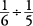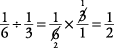Divide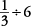.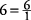, so the problem can be written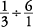. Then invert the second fraction and multiply.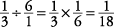Divide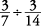.Sometimes, a division of fractions problem may appear in the following form. These are called complex fractions.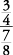The line separating the two fractions means “divided by.” Therefore, this problem may be rewritten as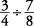Now follow the same procedure as shown in Example .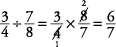Divide =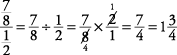To divide mixed numbers, first change them to improper fractions (Example ). Then follow the rule for dividing fractions (Example ).

Divide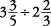Notice that after you invert and have a multiplication of fractions problem, you may then cancel tops with bottoms when appropriate.